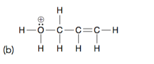Problem: Predict the geometry of each atom except hydrogen in the compounds below:

FREE Expert Solution
85% (444 ratings)
Problem Details

Predict the geometry of each atom except hydrogen in the compounds below:What scientific concept do you need to know in order to solve this problem?

Our tutors have indicated that to solve this problem you will need to apply the Molecular Geometry concept. You can view video lessons to learn Molecular Geometry Or if you need more Molecular Geometry practice, you can also practice Molecular Geometry practice problems .

What is the difficulty of this problem?

Our tutors rated the difficulty of Predict the geometry of each atom except hydrogen in the com... as low difficulty.

How long does this problem take to solve?

Our expert Organic tutor, Jonathan took 2 minutes to solve this problem. You can follow their steps in the video explanation above.

What professor is this problem relevant for?

Based on our data, we think this problem is relevant for Professor Ponnala's class at BMCC CUNY.

What textbook is this problem found in?

Our data indicates that this problem or a close variation was asked in . You can also practice practice problems .